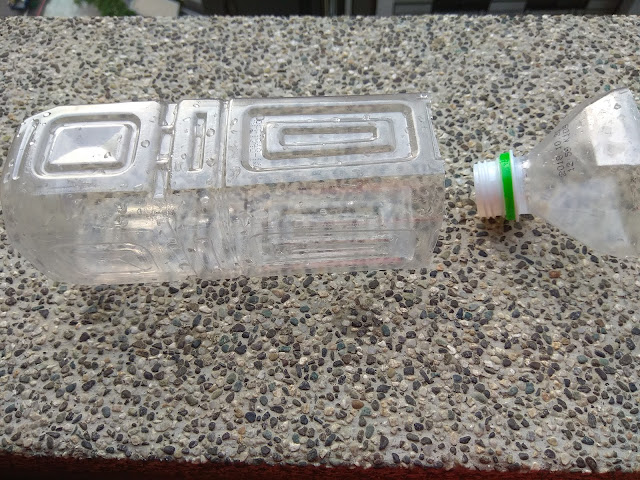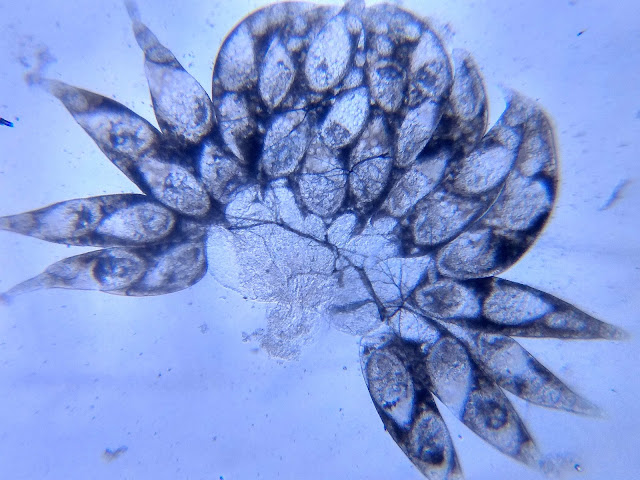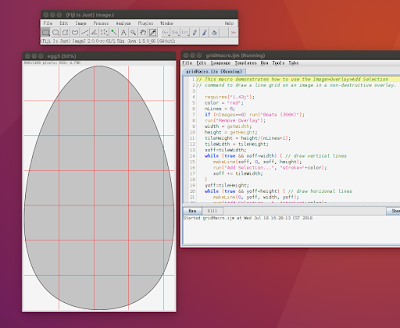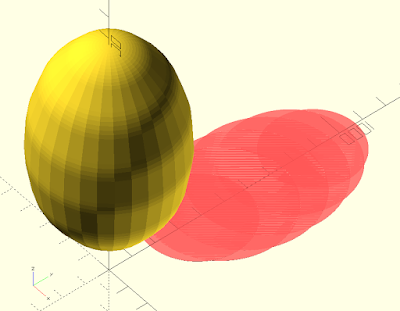## 2018年7月25日

### 用科學Maker社團工具，解剖觀察果蠅卵巢睪丸與精子

1. 我可能找不到二裂果蠅，住家附近能找到的大概是黑腹果蠅(Drosophila melanogaster)，我猜精子大概也很長。
2. 我沒解剖過果蠅，不知道哪裡下刀。而且它睪丸長怎樣啊？
3. 我得抓到果蠅啊## 2018年7月24日

### Imagej配合Weka機器學習plugin做segmentation 偵測葉片氣孔位置 並裁切出單圖for (i=0; i<roiManager("count"); ++i) {
roiManager("Select", i);
run("Duplicate...", "title=crop");
run("Make Inverse");
setForegroundColor(0, 0, 0);
run("Fill", "slice");
saveAs("Tiff", "rticle_"+(i+1)+".tif");
close();
//Next round!
selectWindow("cell.jpg");
}

## 2018年7月18日

### 用imagej加上Macro巨集畫輔助線測量蛋徑requires("1.43j");
color = "red";
nLines = 6;
if (nImages==0) run("Boats (356K)");
run("Remove Overlay");
width = getWidth;
height = getHeight;
tileHeight = height/(nLines+1);
tileWidth = tileHeight;
xoff=tileWidth;
while (true && xoff<width) { // draw vertical lines
makeLine(xoff, 0, xoff, height);
run("Add Selection...", "stroke="+color);
xoff += tileWidth;
}
yoff=tileHeight;
while (true && yoff<height) { // draw horizonal lines
makeLine(0, yoff, width, yoff);
run("Add Selection...", "stroke="+color);
yoff += tileHeight;
}
run("Select None");

### 用openscad畫個蛋r0 = 0;
r1 = 413/2;
r2 = 534/2;
r3 = 567/2;
r4 = 557/2;
r5 = 498/2;
r6 = 372/2;
r7 = 0;
r8 = 0;
r9 = 0;
r10 = 0;

space =100;
trans = max (r0,r1, r2,r3,r4,r5,r6,r7,r8,r9,r10);
bigSqure = 4000;
rotate_extrude(covexity= 10){
intersection(){
square(bigSqure);
rotate([0,0,90])
translate([trans,0,0])
hull(){
translate ([space*0,0,0])  circle(r0,center = true,\$fn = 100);
translate ([space*1,0,0])  circle(r1,center = true,\$fn = 100);
translate ([space*2,0,0])  circle(r2,center = true,\$fn = 100);
translate ([space*3,0,0])  circle(r3,center = true,\$fn = 100);
translate ([space*4,0,0])  circle(r4,center = true,\$fn = 100);
translate ([space*5,0,0])  circle(r5,center = true,\$fn = 100);
translate ([space*6,0,0])  circle(r6,center = true,\$fn = 100);
translate ([space*7,0,0])  circle(r7,center = true,\$fn = 100);
translate ([space*8,0,0])  circle(r8,center = true,\$fn = 100);
translate ([space*9,0,0])  circle(r9,center = true,\$fn = 100);
translate ([space*10,0,0])  circle(r10,center = true,\$fn = 100);

}
}
}